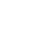# 前言

EC给写操作带来了很大的额外开销，包括编解码计算开销和流程性开销两部分。在向量指令集SSE、AVX等的帮助下，一个现代CPU核心的EC编解码能力就可以达到几GB到十几GB每秒，远远大于存储设备的I/O吞吐率。这使得流程性开销成为EC写入性能的最重要制约因素。若一次写操作的偏移和长度没有对齐EC组，就需要部分更新涉及的EC组，因而将此类操作称为部分写。部分写带来了大部分的流程性开销。

# 投机性部分写：原理、设计与实现

$$\Delta{p_j} = a_{ij} \times \Delta{d_i}$$

$$p_j^{(r)} = p_j^{(0)} + \sum\limits_{x=1}^{r}\Delta p_j^{(x)} = p_j^{(0)} + \sum\limits_{x=1}^{r}a_{ij}(d_i^{(x)} - d_i^{(x-1)}) = p_j^{(0)}+a_{ij} \times (d_i^{(r)}-d_i^{(0)})$$# 实验

### EC编解码性能### PBS部分写的性能### 故障恢复